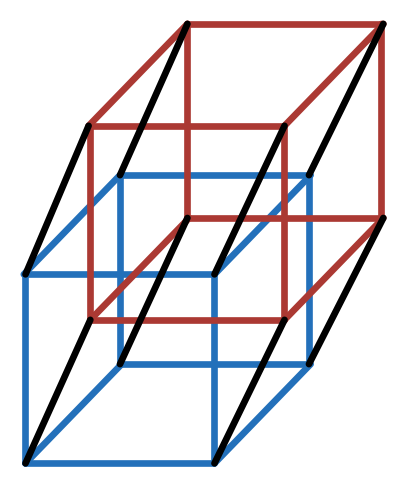# Think 3-D Is Cool? Wait Till You Try 4-D!A lattice hypercube is a cube in 4-dimensional space, whose vertices are lattice points. Describe the set of possible side lengths of a lattice hypercube.

Note: A lattice point is a point whose coordinates are all integers. $(0,0,1)$ is a lattice point, but $\left(\frac{1}{2}, \sqrt{2}, -0.2 \right)$ is not a lattice point.

If you are interested in Lattice cubes, try the 3-D version.

For similar problems, you can read my note on Construction.

×